Next: Deriving a ranking function Up: Probabilistic information retrieval Previous: The PRP with retrieval   Contents   Index

# The Binary Independence Model

The Binary Independence Model (BIM) we present in this section is the model that has traditionally been used with the PRP. It introduces some simple assumptions, which make estimating the probability function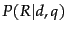practical. Here, binary'' is equivalent to Boolean: documents and queries are both represented as binary term incidence vectors. That is, a document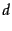is represented by the vector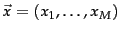where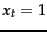if term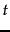is present in documentand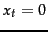ifis not present in. With this representation, many possible documents have the same vector representation. Similarly, we represent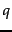by the incidence vector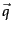(the distinction betweenandis less central since commonlyis in the form of a set of words). Independence'' means that terms are modeled as occurring in documents independently. The model recognizes no association between terms. This assumption is far from correct, but it nevertheless often gives satisfactory results in practice; it is the naive'' assumption of Naive Bayes models, discussed further in Section 13.4 (page). Indeed, the Binary Independence Model is exactly the same as the multivariate Bernoulli Naive Bayes model presented in Section 13.3 (page). In a sense this assumption is equivalent to an assumption of the vector space model, where each term is a dimension that is orthogonal to all other terms.

We will first present a model which assumes that the user has a single step information need. As discussed in Chapter 9 , seeing a range of results might let the user refine their information need. Fortunately, as mentioned there, it is straightforward to extend the Binary Independence Model so as to provide a framework for relevance feedback, and we present this model in Section 11.3.4 .

To make a probabilistic retrieval strategy precise, we need to estimate how terms in documents contribute to relevance, specifically, we wish to know how term frequency, document frequency, document length, and other statistics that we can compute influence judgments about document relevance, and how they can be reasonably combined to estimate the probability of document relevance. We then order documents by decreasing estimated probability of relevance.

We assume here that the relevance of each document is independent of the relevance of other documents. As we noted in Section 8.5.1 (page), this is incorrect: the assumption is especially harmful in practice if it allows a system to return duplicate or near duplicate documents. Under the BIM, we model the probabilitythat a document is relevant via the probability in terms of term incidence vectors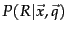. Then, using Bayes rule, we have: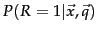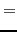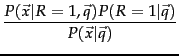(63)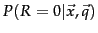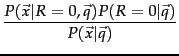(64)

Here,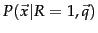and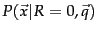are the probability that if a relevant or nonrelevant, respectively, document is retrieved, then that document's representation is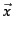. You should think of this quantity as defined with respect to a space of possible documents in a domain. How do we compute all these probabilities? We never know the exact probabilities, and so we have to use estimates: Statistics about the actual document collection are used to estimate these probabilities.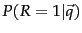and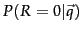indicate the prior probability of retrieving a relevant or nonrelevant document respectively for a query. Again, if we knew the percentage of relevant documents in the collection, then we could use this number to estimateand. Since a document is either relevant or nonrelevant to a query, we must have that: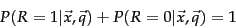(65)

SubsectionsNext: Deriving a ranking function Up: Probabilistic information retrieval Previous: The PRP with retrieval   Contents   Index